# 常见的五种神经网络(3)-循环神经网络（中）篇

Github：https://github.com/thinkgamer

• 循环神经网络中的参数学习
• RNN中的长期依赖问题
• 常见的循环神经网络结构

### 参数学习

$L_t = L(y_t, g(h_t))$

$L = \sum_{t=1}^{T} L_t$

$\frac{\partial L}{\partial U} = \sum _{t=1}^{T}\frac{\partial L_t}{ \partial U }$

• 随时间反向传播算法（Backpropagation Through Time，BRTT）
• 实时循环学习（Real-Time Recurrent Learning，RTRL）

#### 随时间反向传播算法

$\frac{\partial L_t}{ \partial U_{ij}} = \sum_{k=1}^{t} tr( ( \frac{\partial L_t}{ \partial z_k} )^T \frac{\partial^+ z_k}{ \partial U_{ij}} ) \\ = \sum_{k=1}^{t} ( \frac{\partial^+ z_k}{ \partial U_{ij}} )^T \frac{\partial L_t}{ \partial z_k}$

$\frac{\partial^+ z_k}{ \partial U_{ij}} = \begin{bmatrix} 0\\ ... \\ [h_{k-1}]_j \\ ... \\ 0 \end{bmatrix} \triangleq I_i([h_{k-1}]_j)$

$\delta _{t,k} = \frac{\partial L_t}{ \partial z_k } \\ = \frac{ \partial h_k }{ \partial z_k} \frac{\partial z_{k+1}}{ \partial h_k } \frac{ \partial L_t }{ \partial z_{k+1} } \\ = diag(f'(z_k))U^T \delta _{t,k+1}$

$\frac{\partial L_t}{ \partial U_{ij} } = \sum_{k=1}^{ t } [\delta _{t,k}]_i [h_{k-1}]_j$

$\frac{\partial L}{ \partial U } = \sum_{k=1}^{ t } \delta _{t,k} h^T_{k-1}$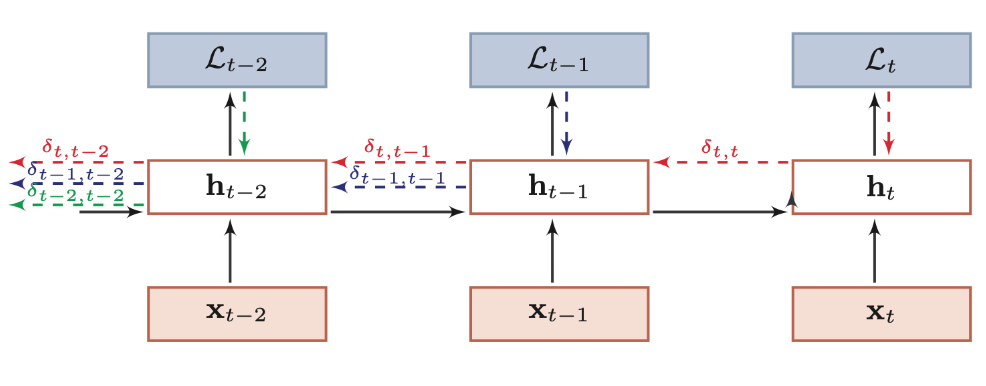$\frac{\partial L}{ \partial U } = \sum_{t=1}^{ T}\sum_{k=1}^{ t } \delta _{t,k} h^T_{k-1}$

$\frac{\partial L}{ \partial W } = \sum_{t=1}^{ T}\sum_{k=1}^{ t } \delta _{t,k} x^T_k$

$L$关于参数$b$的梯度（公式1-11）：
$\frac{\partial L}{ \partial b } = \sum_{t=1}^{ T}\sum_{k=1}^{ t } \delta _{t,k}$

#### 实时循环学习

$h_{t+1} = f(z_{t+1}) = f(Uh_k + Wx_{k+1} + b)$

$\frac{ h_{t+1} }{ \partial U_{ij} } = \frac{ \partial h_{t+1} }{ \partial z_{t+1} } ( \frac{ \partial^+z_{t+1} }{ \partial U_{ij}} + U \frac{ \partial h_t}{ \partial U_{ij} } ) \\ = diag( f'(z_{t+1}) ) ( I_i ([h_t]_j)+ U \frac{ \partial h_t}{ \partial U_{ij} } ) \\ =f'(z_{t+1}) \odot ( I_i ([h_t]_j)+ U \frac{ \partial h_t}{ \partial U_{ij} } )$

RTRL自从第一个时刻开始，除了计算RNN的隐状态之外，还利用（公式1-13）依次前向计算偏导数$\frac{\partial h_1}{ \partial U_{ij}},\frac{\partial h_2}{ \partial U_{ij}},\frac{\partial h_3}{ \partial U_{ij}}...$

$\frac{\partial L_t}{ \partial U_{ij}} =( \frac{\partial h_t}{ \partial U_{ij} } )^T \frac{\partial L_t}{ \partial h_t}$

### 长期依赖

$\delta _{t,k}=\prod_{i=k}^{t-1} ( diag('f(z_i ))U^T )\delta _{t,t}$

$\delta _{t,k}=\gamma ^{t-k} \delta _{t,t}$
$\gamma >1$，当$t-k \rightarrow +\infty$$\gamma ^{t-k} \rightarrow +\infty$，会造成系统不稳定，称之为梯度爆炸（Gradient Exploding Problem），反之，若$\gamma < 1$，当$t-k \rightarrow +\infty$$\gamma ^{t-k} \rightarrow 0$，会出现和前馈神经网络类似的梯度消失问题（Gradient Vanishing Problem）。

• 选取合适的参数
• 使用非饱和的激活函数

### 常见的循环神经网络结构

• N：N
• 1：N
• N：1
• N：M

#### N比N结构

N维输入对应N维输出，大致结构如下所示：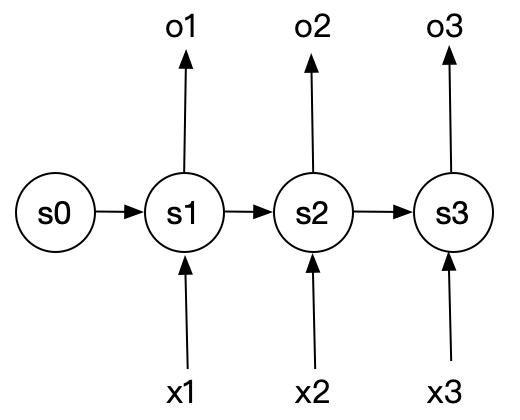• 视频理解中获取视频每一帧标签，输入为视频解码后的图像，通过此结构，获取每一 帧的标签信息。这种场景一般用作视频理解的初期，对视频做初步的处理后， 后续可以基于这些标签信息进行语义分析，构建更为复杂的需求场景。
• 股票价格预测。基于历史的股票信息输入，预测下一时刻或者未来的股票走势信息。

#### 1比N结构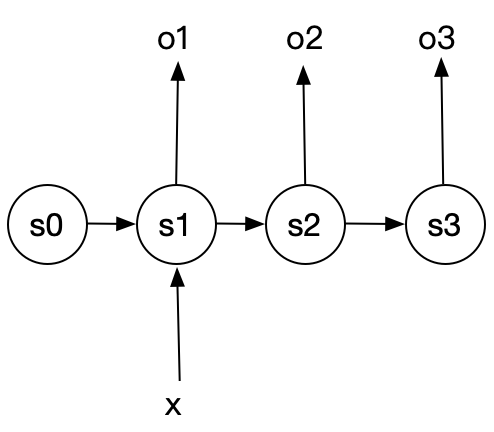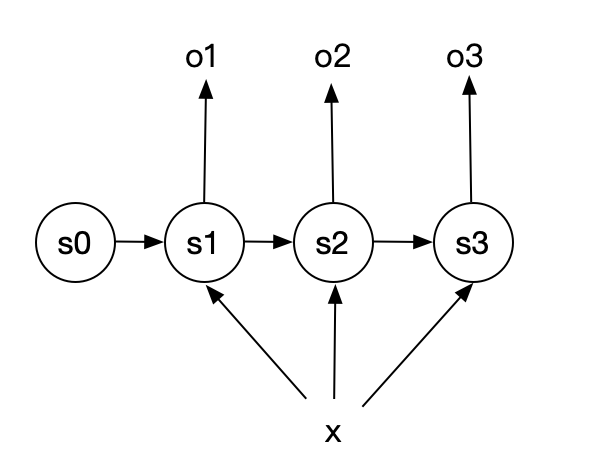• 看图写描述 : 根据输入的 一张图 片，生成对这张图片的描述信息
• 自动作曲 : 按照类别生成音乐

#### N比1结构

N维输入，一维输出，大致结构如下图所示：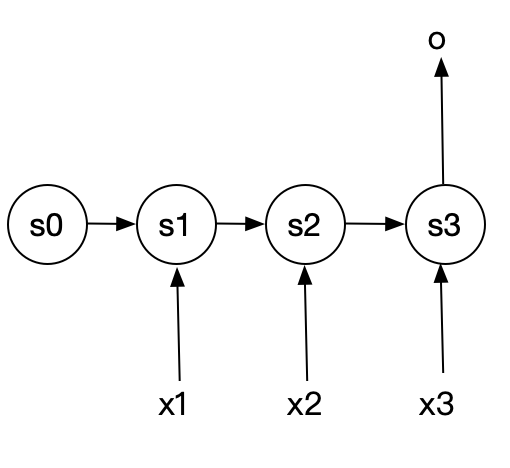• 视频理解中的获取视频每个场景的描述信息，或者获取整个影片的摘要信息。
• 获取用户评价的情感信息，即根据用户的一句话的评论，来判断用户的喜好等情感信息 。

#### N比M结构

N维输入，M维输出，这种结构又被称为Encoder-Decoder模型，也可以称为Seq2Seq模型，这种模型的输入和输出可以不相等，该模型由两部分组成：编码部分和解码部分，大致结构如下图所示：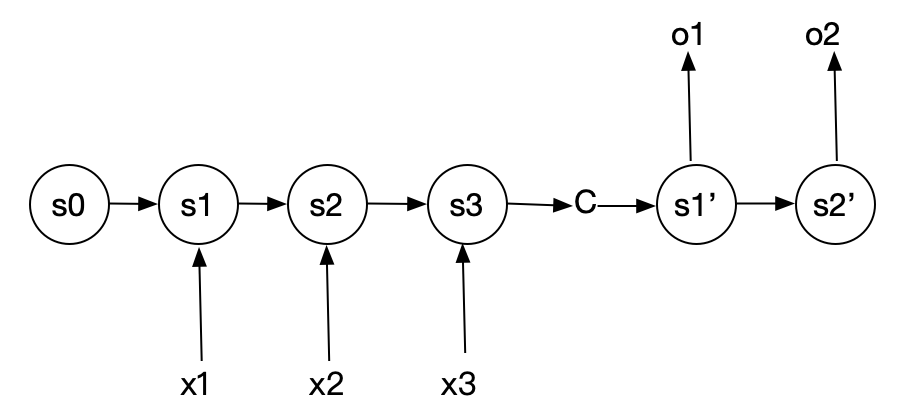c的前半部分循环神经网络为编码部分，称之为Endcoder, c可以是s3的直接输出，或者 是对s3输出做一定的变换，也可以对编码部分所有的s1、s2、s3进行变换得到，这样c中就包 含了对X1、 Xz、码的编码信息 。c的后半部分循环神经网络为解码部分，称之为Decoder。c作为之前的状态编码，作为初始值，输入到Decoder当中。 Decoder经过循环处理，最终将信息解码输出。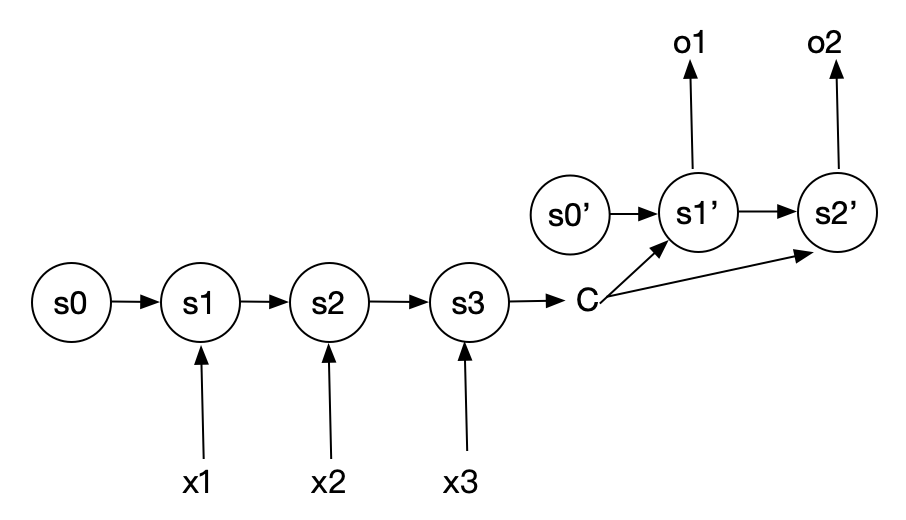N比M的循环神经网络结构更具有普遍性，现实环境中有很多基于该结构落地的场景，他可以解决如下问题：

• 机器翻译：将不同语言作为输入，输出为非输入语言的类型，这也是Encoder-Decoder的经典用法
• 文本摘要：输入一篇文章，输出这篇文章的摘要信息
• 语音识别：输入一段语音，输出这段语音信息的文字【搜索与推荐Wiki】专注于搜索和推荐系统，尝试使用算法去更好的服务于用户，包括但不局限于机器学习，深度学习，强化学习，自然语言理解，知识图谱，还不定时分享技术，资料，思考等文章！